SYLLABUS  Previous: 4.1.1 The European Black-Scholes  Up: 4.1 Plain vanilla stock  Next: 4.1.3 Application, time value

4.1.2 Parameters illustrated with VMARKET experiments

[ SLIDE parameters - volatility - rate - dividend || VIDEO modem - LAN - DSL ]

Apart from the terminal conditions that specify the value of an option when it expires (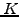for a vanilla option or StrikePrice in the applet) and the numerical parameters that specify the precision of the calculation (such as the TimeStep, Walkers and MeshPoints), the Black-Scholes model depends on only four financial parameters:

• The time to the expiry (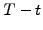or RunTime) is usually expressed in a fraction of a year, e.g. 0.25 for three months or a quarter of year to the expiry date.
• The short term interest or spot rate (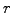or SpotRate) is specified as a annual fraction of the capital investment, e.g. 0.05 for a risk free return of five percent per year.
• the dividend yield (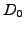or Dividend), here modeled with a continuous payment that is proportional to the underlying price, e.g. 0.04 for a dividend paying four percent of the share value during one year,
• the volatility of the underlying (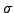or Volatility) estimated as the standard deviation of closing prices in sect.1.5, e.g. 0.5 for 50 percent for a volatile share.
To clearly visualize the payoff dynamics from the Black-Scholes model, it is useful to increase the interest rate and the dividend yield to artificially large values and study with the VMARKET applet below how the parameters affect the price of a European call struck for 10 EUR six months before expiry.

The evolution of the payoff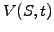should be far from obvious, but can be disentangled by investigating the effect of each parameter separately.

Have a look first at the volatility using the VMARKET applet above.

 Virtual market experiments: volatility Set SpotRate=0, Dividend=0 and observe how the price changes as the time runs backwards, i.e. for an increasing amount of time to the expiry date. Click inside the plot area and use the coordinates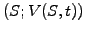displayed in the browser status field and the Java console to measure the price of the call at-the-money as a function of time. You can use Step 10 to simulate an evolution backwards with multiples of ten days and collect the quantitative data on a sheet of paper. How do the prices move in reality when the time runs forward and approaches the expiry date? Run the applet with a fixed time to expiry (e.g. RunTime=0.5) and measure the price for a Volatility ranging from zero to about one. How does the price of the option at-the-money depend on the volatility? Repeat both experiments after switching to a Put option.

These experiments show that the main effect of the volatility is to smear out sharp edges'', i.e. where the vanilla call and put options are at-the-money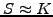. This phenomenon, known as diffusion in engineering sciences, is strongest at the begining of the simulation when the option is close to expiry date. It is the result of unpredictable market fluctuations: even if the value of the underlying share is below the strike price of a call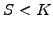before the expiry date, there is a finite chance that the market price will suddenly rise above that value, which would allow the holder of a call option to make a final profit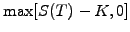. Such a right to make a potential profit without any obligation has of course a finite value, which decreases as the time approaches the expiry date.

Now play with the VMARKET applet below, using the default parameters focusing on the effect of the interest rate.

 Virtual market experiments: interest rate Observe how the prices change with an increasing lifetimefor the option. How do the prices move in reality when the calendar time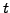runs forward? Run the applet with a fixed option lifetime (e.g. RunTime=0.5) and measure the price of a put option deeply in-the-money as a function of the SpotRate parameter. What happens to the payoff when the underlying share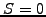, i.e. the company goes bankrupt? Why? Repeat the experiments after switching to a Call option and explain the qualitative difference in financial terms.

The effect of the risk-free interest rate can be understood from the drift that affects any type of investment: to finally coincide with the exercise priceon the expiry date, the strike price has to be discounted back in time (1.3#eq.6) to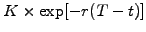. This is clearly visible in the applet, where the value at-the-money shifts to lower prices as the simulation runs backward in time. With a drift that is proportional to the strike price, the interest rate appears to have its largest relative effect when the option is at-the-money while the underlying is kept fixed; this is somewhat misleading, since the underlying should also grow by the same amount but is here used as a parameter. In fact, the graph could be continuously renormalized with the same amplification factor for the share, strike and option value-e.g. introducing a new currency after every time step, so that the graph would not evolve anymore at all.

The following experiments can be carried out in the VMARKET applet above.

 Virtual market experiments: dividend yield Set the SpotRate=0 and study the payoff from a call option when the underlying pays a Dividend=0.4; compare with the effect from an interest rate. Repeat the experiment switching to a Put option; explain why the payoff V(0,t) remains constant for an underlying company that goes bankrupt (S=0). Set SpotRate=Dividend=0.4 and try to explain what happens when switching to both Call and Put options.

Hopefully, these last experiments contribute more to your understanding than your confusion: with payments that are proportional to the underlying, the dividend yield continuously reduces the value of the share by the same amount; this results in a drift along the horizontal axis (in the opposite direction from the effect of interest rates) and appears as if the share prices were amplified when the time runs backwards. If the interest rate is equal to the dividend yield, the drifts in the horizontal direction cancel out and all that remains is the effect from the discounting at a risk-free interest rate.

With a good intuition for each parameter taken separately, it is a good exercise to now return to the first applet and discuss the main features that characterize an option payoff when all the parameters are combined into one calculation. Also, remember that unrealistically large parameters have been used in this section to exaggerate the effect from each parameter; realistic values will be used for an real option pricing calculation in the next section.

SYLLABUS  Previous: 4.1.1 The European Black-Scholes  Up: 4.1 Plain vanilla stock  Next: 4.1.3 Application, time value